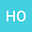On a conjecture of Davies and Levitin
•## Abstract

Let $H_c$ be a $(2n)\times(2n)$ symmetric tridiagonal matrix with diagonal elements $c \in \mathbb{R}$ and off-diagonal elements one, and $S$ be a $(2n)\times(2n)$ diagonal matrix with the first $n$ diagonal elements being plus ones and the last $n$ being minus ones. Davies and Levitin studied the eigenvalues of a linear pencil $\mathcal{A}_c=H_c-\lambda S$ as $2n$ approaches to infinity. It was conjectured by DL that for any $n \in \mathbb{N}$ the non-real eigenvalues $\lambda$ of $\mathcal{A}_c$ satisfy both $|\lambda + c|<2$ and $|\lambda - c|<2$. The conjecture has been verified numerically for a wide range of $n$ and $c$, but so far the full proof is missing. The purpose of the paper is to support this conjecture with a partial proof and several numerical experiments which allow to get some insight in the behaviour of the non-real eigenvalues of $\mathcal{A}_c$. We provide a proof of the conjecture for $n \leq 3$, and also in the case where $|\lambda + c|=|\lambda - c|$. In addition, numerics indicate that some phenomena may occur for more general linear pencils.
03 Feb 2022Submitted to Mathematical Methods in the Applied Sciences
04 Feb 2022Submission Checks Completed
04 Feb 2022Assigned to Editor
18 Mar 2022Reviewer(s) Assigned
02 Aug 2022Review(s) Completed, Editorial Evaluation Pending
02 Aug 2022Editorial Decision: Revise Major